Courses

# Properties Of Gasses And Gas Mixture - MCQ Test 1

## 20 Questions MCQ Test Mock Test Series - Mechanical Engineering (ME) for GATE 2020 | Properties Of Gasses And Gas Mixture - MCQ Test 1

Description
This mock test of Properties Of Gasses And Gas Mixture - MCQ Test 1 for Mechanical Engineering helps you for every Mechanical Engineering entrance exam. This contains 20 Multiple Choice Questions for Mechanical Engineering Properties Of Gasses And Gas Mixture - MCQ Test 1 (mcq) to study with solutions a complete question bank. The solved questions answers in this Properties Of Gasses And Gas Mixture - MCQ Test 1 quiz give you a good mix of easy questions and tough questions. Mechanical Engineering students definitely take this Properties Of Gasses And Gas Mixture - MCQ Test 1 exercise for a better result in the exam. You can find other Properties Of Gasses And Gas Mixture - MCQ Test 1 extra questions, long questions & short questions for Mechanical Engineering on EduRev as well by searching above.
QUESTION: 1

### A mono-atomic ideal l gas (γ = 1.67, molecular weight = 40) is compressed adiabatically from 0.1 MPa, 300 K to 0.2 MPa. The universal gas constant is 8.314 kJ kmol-1K-1. The work of compression of the gas (in kJ kg-1) is:

Solution: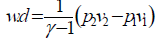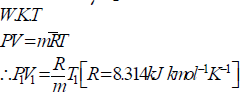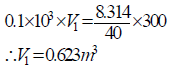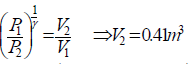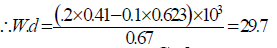QUESTION: 2

Solution:
QUESTION: 3

### A frictionless piston-cylinder device contains a gas initially at 0.8 MPa and 0.015 m3. It expands quasi-statically at constant temperature to a final volume of 0.030 m3. The work output (in id) during this process will be:

Solution:

Iso-thermal work done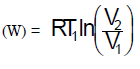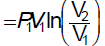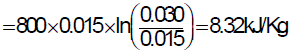QUESTION: 4

Assertion (A): The mass flow rate through a compressor for various refrigerants at same temperature and pressure, is proportional to their molecular weights.

Reason (R): According to Avogardo‟s Law all gases have same number of moles in a given volume of same pressure and temperature.

Solution:
QUESTION: 5

In a reversible isothermal expansion process, the fluid expands from 10 bar and 2 m3 to 2 bar and 10m3, during the process the heat supplied is 100 kW. What is the work done during the process?

Solution:

As internal energy is a function of temperature only. In isothermal expansion process no temperature change therefore no internal energy change. A Reversible isothermal expansion process is constant internal energy process i.e. dU = 0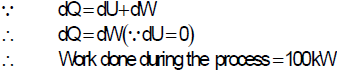QUESTION: 6

Which of the following statement is correct?

Solution:

True. A water film, if formed, will act as a very poor conductor of heat and will not easily let the heat of the furnace pass into the boiler. An oil film if present, is even worse than water film and the formation of such films inside the boiler must be avoided.
(b) Since the mass and material are the same, the volumes must also be the same. For the same volume, the surface area of the plate is the greatest and that of the sphere is
the least. The rate of loss of heat by radiation being proportional to the surface area, the plate cools the fastest and the sphere the slowest.

(c) True, for a monoatomic gas,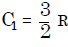and for a diatomic gas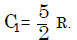Since the mixture has two moles, the value of C1 for the mixture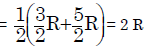(d) False, The average kinetic energy of 1 g of an ideal gas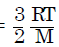Where M is the molecular weight of the gas and it is different gases, as the value of Mb will be different.

QUESTION: 7

The correct sequence of the decreasing order of the value of characteristic gas constants of the given gases is:

Solution:

The correct sequence for decreasing order of the value of characteristic gas constants is hydrogen, nitrogen, air and carbon dioxide.

QUESTION: 8

Pressure reaches a value of absolute zero

Solution:

we know that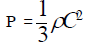If momentum is zero then C must be zero. Hence P would be zero. That will occur at absolute zero temperature. But note here choice (a) has in defined temp. –273K which is imaginary temp.

QUESTION: 9

Which of the following statement about Van der waal's equation i valid?

Solution:
QUESTION: 10

Consider the following statements:

1. A gas with a compressibility factor more than 1 is more compressible than a perfect gas.

2. The x and y axes of the compressibility chart are compressibility factor on y-axis and reduced pressure on x-axis.

3. The first and second derivatives of the pressure with respect to volume at critical points are zero.

Which of the statements given above is/are correct?

Solution:

1 is false. At very low pressure, all the gases shown have z ≈1 and behave nearly perfectly. At high pressure all the gases have z > 1, signifying that they are more difficult
to compress than a perfect gas (for a given molar volume, the product pv is greater than RT). Repulsive forces are now dominant. At intermediate pressure, must gasses have Z < 1, including that the attractive forces are dominant and favour compression.

QUESTION: 11

Assertion (A): At very high densities, compressibility of a real gas is less than one.

Reason (R): As the temperature is considerably reduced, the molecules are brought closer together and thermonuclear attractive forces become greater at pressures around 4 MPa.

Solution:
QUESTION: 12

Assertion (A): The value of compressibility factor, Z approaches zero of all isotherms as pressure p approaches zero.

Reason (R): The value of Z at the critical points is about 0.29.

Solution:
QUESTION: 13

A control mass undergoes a process from state 1 to state 2 as shown in the given figure. During this process, the heat transfer to the system is 200 KJ. IF the control mass returned adiabatically. From state 2 to state 1 by another process, then the work interaction during the return process (in kNm) would be: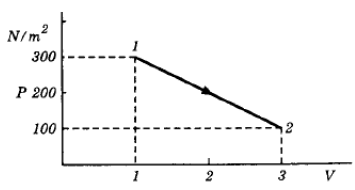Solution:

done = change in internal energy.
Since control man (so case of closed
system). Intercept of path on X-axis
is the work done by the process.

0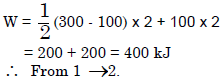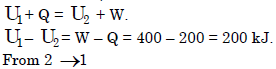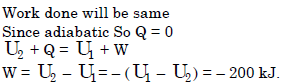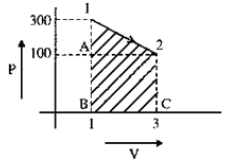QUESTION: 14

A balloon which is initially collapsed and flat is slowly filled with a gas at 100 kPa so as to form it into a sphere of 1 m radius. What is the work done by the gas in the balloon during the filling process?

Solution:

Work done by the gas during filling process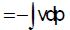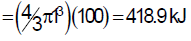QUESTION: 15

This process is one in which there is only work interaction between the system and the Surroundings

Solution:
QUESTION: 16

The work done in compressing a gas isothermally is given by

Solution:
QUESTION: 17

The work done during expansion of a gas is independent of pressure if the expansion takes place

Solution:
QUESTION: 18

Three moles of an ideal gas are compressed to half the initial volume at a constant temperature of 300k. The work done in the process is

Solution:

Since the temperature remains constant, the process is isothemal.

Work-done in the process,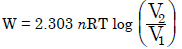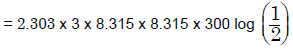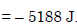QUESTION: 19

Assertion (A): Though head is added during a polytropic expansion process for which γ > n> 1, the temperature of the gas decreases during the process.

Reason (R): The work done by the system exceeds the heat added to the system.

Solution:
QUESTION: 20

The heat absorbed or rejected during a polytropic process is equal to:

Solution: